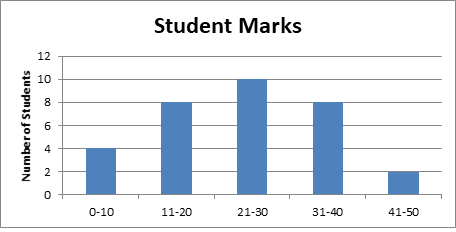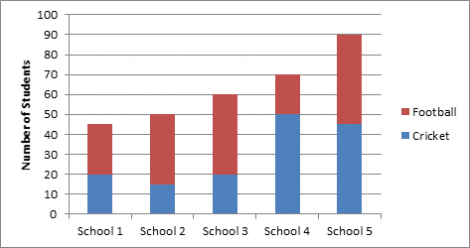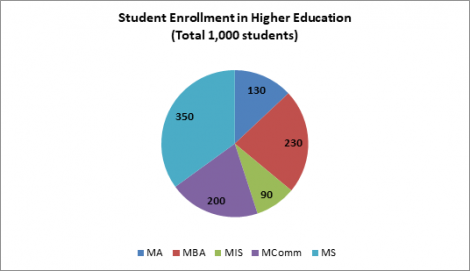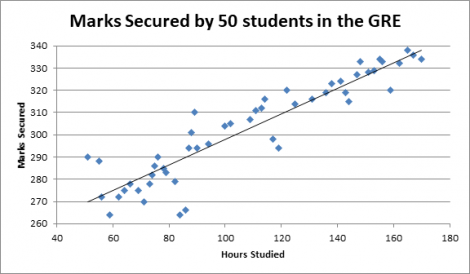# The Definitive GRE Data Interpretation GuideThe GRE Quant section tests students on four areas: Arithmetic, Algebra, Geometry and Data Analysis. To master the first four types of questions – knowing concepts and how to apply them is enough. But Data Interpretation type questions (i.e. Data Analysis) are special. They require the skill to:

1. Understand the data given in graphical or tabular format
2. Understand how the questions asked link to the data in the graph / table
3. Convert the question into a mathematical equation
4. Fetch the relevant data from the graph / table to solve the equation and get the answer

It’s important to master these skills because roughly 15%-20% (i.e. 6 to 8 questions) on the GRE Quant Section will be of this type.

Data Interpretation set question types are based on 6 different ways of representing data. So the key to success in this section is to understand the different data representation types. This guide describes the data representation types tested in the GRE Data Analysis and provides you with strategies to tackle this section. So here we go!

Frequency Distribution Tables

Frequency Distribution Tables typically are 2 column tables in which categories or numerical values of data are listed in the first column and the corresponding frequencies are listed in the second column. A variant of this is the relative frequency distribution table which has the same layout but with relative frequencies recorded for each data category instead of the actual frequency. This is done by using percentages. Consider the following sample frequency table and relative frequency table that represent the same data i.e. scores obtained by a number of children in a class who have taken a Math Test:

Frequency Table

 Marks Frequency (No. of Students) 0-10 4 11-20 8 21-30 10 31-40 8 41-50 2

Relative Frequency Table

 Marks Relative Frequency (Percentage  of Students) 0-10 12.5% 11-20 25% 21-30 31.25% 31-40 25% 41-50 6.25%

Note that in the relative frequency table, summing the percentages adds up to 100%. This is because taken together; the categories cover the entire set of students.

Bar Graphs

Data from frequency distribution tables can also be represented in bar charts. In such charts, each of the data categories or numerical values is represented by a rectangular bar and the height of each bar is proportional to the corresponding frequency or relative frequency. The bars can be drawn either vertically or horizontally. Bar graphs enable comparisons across several categories more easily than tables. To illustrate this point here’s an example of a bar chart for the same data which was represented as a frequency distribution table in the previous section:From this chart, it’s very easy to see that the number of students who secured between 21 and 30 is the most and the number of students who secured between 41 and 50 is the least.

Stacked Bar Graphs

A stacked (or segmented) bar graph is similar to a regular bar graph except that in a stacked bar graph, each rectangular bar is divided, or segmented into smaller rectangles that show how the variable is “divided” into other related variables. For example, consider the following:

The bar graph below shows the number of students playing sport at five schools A, B, C, D and E. Each student represented in the bar graph below plays either cricket or football.In the stacked bar graph above, the red rectangles represent the number of students playing football and the blue rectangles represent the number of students playing cricket. Observe that the number of students playing sport in School 5 (90 students) is the most and is the least in School 1 (45 students). However, note that the largest number of students playing cricket is in School 4 (50 students). Such representation of data is more concise than creating two separate bar graphs for football and cricket

Pie Charts

Pie Charts are used to show data that have been separated into a small number of categories. The data are presented in a circle such that the area of the circle representing each category is proportional to the part of the whole that the category represents. An example of a pie chart is given below:Each part of the circle is called a sector. The ratio of each sector’s area to the total area of the pie chart is equal to the ratio of the measure of it’s central angle to 360 degrees. For example the ratio of the area of the sector representing students who pursue MComm is 200/1000 i.e. 0.2 or 20% of the pie chart’ area. This ratio is equal to the measure of the central angle subtended by the MComm sector to 360 degrees. So the measure of the central angle of this sector will be 20% X 360 degrees or 72 degrees.

Scatter Plots

A scatterplot is a type of graph that shows the relationship between numerical variables whose values can be observed in a single population of individual objects. In a scatterplot, for each object, the value of one variable appears on the horizontal axis and the corresponding value of the other variable for that same object appear on the vertical axis. The two values for each object form an ordered pair and this pair is represented by a point in the coordinate system.

Consider the following example:

A researcher was studying the correlation between hours studied for the GRE and the marks obtained. For this purpose, she interviewed 50 students and plotted their results on a scatterplot as shown below.When a trend line is included in the scatterplot, you can observe how scattered or close the data are to the trend line. In the scatterplot above, most of the data points are relatively close to the trend line. In general, one can make the conclusion that the more number of hours that you study, the higher the marks that you secure on the GRE.

Such trend lines can be used to make predictions. For example, it can be predicted from the graph above that someone who studies for 150 hours is likely to score roughly 320 marks. Another useful prediction that can be made is how many incremental marks one could score by studying for 1 extra hour. This can be found by finding the ratio of change in the marks secured to the change in hours studied – or in other words, the slope of the line. To estimate the slope of any two points on the line:

1. First estimate the coordinates of the two points
2. Then find the difference between the vertical and horizontal coordinate values of both points
3. Finally divide the difference of the vertical and horizontal values of coordinates

For example the slope between the extreme left and right points in the graph i.e. (50, 290) & (170, 334) can be given by the value (334-290)/(170-50) i.e. 0.36. This implies that for every 1 extra hour of study, students can expect a 0.36 increase in their GRE score. The slope in this case will have the unit incremental marks per hour studied i.e the slope is 0.36 incremental marks/hour studied. So, theoretically, one would need to study approximately for 3 extra hours to increase their GRE score by 1 mark.

Line Graphs

A line graph is another type of graph that is that is useful for showing the relationship between two numerical variables. A line graph uses a coordinate plane where each data point represents a pair of values observed for the two numerical variables. For example, the line graph below shows how the population of a town increased over a period of 10 years:The line graph above shows that the greatest increase in population between consecutive years was the change from 2018 to 2019. One way to determine this is by noting that the slope of the line segment joining the values for 2018 and 2019 is greater than the slopes of the line segments joining all other consecutive years. Another way to determine this is to note that the change in population between 2018 and 2019 was greater than 5,000 but was less than 5,000 for all the preceding years.

Though the GRE will test you on several question types in data interpretation; the graphs that you see will be variations of those explained above. Now that you are aware of the types of data representation that you might encounter in the GRE, let’s take a look at some strategies to tackle them:

Typically, GRE data interpretation questions appear in sets. When this happens, there’s usually a “question stem” i.e. a written introduction of the data based on which linked questions are asked. It’s a good idea to read the question stem before looking at the data table / graph as this sets the right context. This technique helps reduce the time taken attempt the linked questions as you will know exactly what to look for when interpreting the graph / table. Without this, you’d end up reading the graph / table, then reading the questions and then again reading the graph to fetch the right data to solve the question.

One of skills needed to perform well in any Master’s program is to have attention to detail. This is tested frequently in data interpretation questions. Often, the GRE will trick students by asking questions in one system of units but representing the graph in another system of units. So students need to read the dimensions of the x and y axes of graphs carefully.

Another area that students often make mistakes in is assuming information. For example, if the question states that Student A’s marks increased from the 1st to the 2nd test by 30% and Student B’s marks decreased by 40%, the natural assumption would be to conclude that Student A did better than Student B in the 2nd test. This may not be true – since the question is stating facts in terms of percentages – not absolute values. So don’t assume anything that isn’t explicitly mentioned in the question.

Use estimation effectively

In the GRE quant section, students have roughly 1.5 minutes to solve a question. This isn’t much and pacing yourself is critical. One skill that helps with getting the right answer quick – especially in data interpretation type questions is using estimation to solve questions. This is not something that comes naturally – but rather requires consistent practice. But once you get the hang of it, it actually simplifies things a lot.

For example, knowing that 52% of 600 should be close to 300 is powerful – because the answer options might be spread out in such a manner that you don’t actually have to calculate the exact value thereby saving precious time.

Take notes

When you’re reading the question stem, write down what’s being asked. Then, when you start interpreting the data in the graph / table – do write down important values – like data points at the extremes, the units etc. While this might increase the time taken to solve the question, it will help you make sense of the data clearly. This in turn improves your odds of arriving at the right answer.

That’s a wrap on our guide to the GRE data interpretation guide!

If you liked it, feel free to share this guide on social media. If you have any additional tips that might help students, let us know in the comments below.